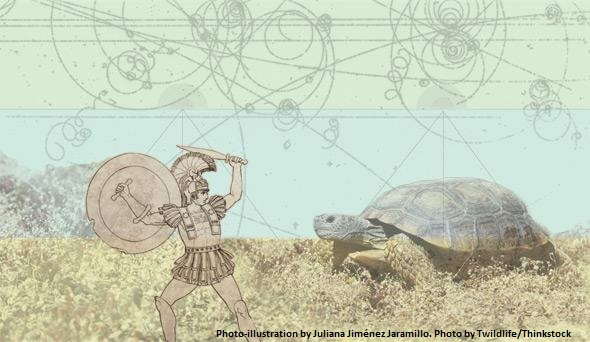# MTH321: Real Analysis I (Spring 2020)

Discussion is available at the end of this page. One is free to ask any question or comment.At the end of this course the students will be able to understand the basic set theoretic statements and emphasize the proofs’ development of various statements by induction. Define the limit of, a function at a value, a sequence and the Cauchy criterion. Prove various theorems about limits of sequences and functions and emphasize the proofs’ development. Define continuity of a function and uniform continuity of a function, prove various theorems about continuous functions and emphasize the proofs’ development. Define the derivative of a function, prove various theorems about the derivatives of functions and emphasize the proofs’ development. Define a cluster point and an accumulation point, prove the Bolzano-Weierstrass theorem, Rolles’s Theorem, extreme value theorem, and the Mean Value theorem and emphasize the proofs’ development. Define Riemann integral and Riemann sums, prove various theorems about Riemann sums and Riemann integrals and emphasize the proofs’ development.

• The Real Number System: Ordered Fields. The Field of Reals. The Extended Real Number System. Euclidean Space.
• Numerical Sequences and Series. Limit of a Sequence. Bounded Sequences. Monotone Sequences. Limits Superior and Inferior. Subsequences.
• Limit of a Function and Continuous Functions. Uniform Continuity. Kinds of Discontinuities.
• Derivable and Differentiable Functions. Mean Value theorems. The Continuity of Derivatives. Taylor's theorem.
• Riemann Stieltijes Integrals: Definition, Existence and Properties of the Riemann Integrals. Integral and Differentiation.
Did you know?
• The development of calculus in the 18th century used the entire set of real numbers without having defined them cleanly. The first rigorous definition was given by Georg Cantor in 1871.
• In the 16th century, Simon Stevin created the basis for modern decimal notation, and insisted that there is no difference between rational and irrational numbers in this regard.

The classes has been suspended due to COVID-19 pandemic.

• Thursday, 1630-1700, CR-7
• Friday, 1630-1700, CR-7

Please download PDF files of the notes given below. To view PDF files, there must be PDF Reader (Viewer) installed on your PC or mobile or smartphone. It can be downloaded from Software section or a user viewing on Android Smartphone may consider EBookDroid to view PDF on their smartphone.

• Chapter 05: Differentiation | Download PDF• Chapter 06: Riemann Integral (Introduction Only) | Download PDF• Assignment 03 | Download PDF |#### Chapter 02

• 2.01- Define sequence of real numbers.
• 2.02- Define subsequence
• 2.03- Define increasing sequence.
• 2.04- Define decreasing sequence.
• 2.05- Define monotnone sequence.
• 2.06- Define bounded sequence.
• 2.07- Prove that $\{\frac{1}{n}\}$ is decreasing sequence.
• 2.08- Prove that $\{1+\frac{1}{n}\}$ is a decreasing sequence.
• 2.09- Prove that $\{\frac{n+1}{n+2}\}$ is increasing sequence.
• 2.10- Is the sequence $\{\frac{n+2}{n}\}$ is increasing or decreasing sequence?
• 2.11- Define convergence of the sequence.
• 2.12- By definition, prove that $\lim\limits_{n\to\infty}\frac{2n}{n+2}=2$.
• 2.13- By definition, prove that $\lim\limits_{n\to\infty}\frac{n^2-1}{2n^2+3}=\frac{1}{2}$.
• 2.14- By definition, prove that $\lim\limits_{n\to\infty}\frac{1}{3^n}=0$.
• 2.15- Prove that a convergent sequence of real number has one and only one limit.
• 2.16- Prove that the limit of the sequence is unique. (alternative statement of Q # 15)
• 2.17- Prove that if the sequence $\{s_n\}$ converges to $s$, where $s\neq 0$, then there exists a positive integer $n_1$ such that $|s_n|>\frac{1}{2}|s|$ for all $n>n_1$.
• 2.18- Prove that if the sequence $\{s_n\}$ converges to $s$, where $s\neq 0$, then there exists a positive integer $n_1$ such that $|s_n|>\frac{1}{5}|s|$ for all $n>n_1$.
• 2.19- Prove that if $\lim\limits_{n\to\infty}{s_n}=t$, then $\lim\limits_{n\to\infty}{|s_n|}=|t|$ but converse is not true in general.
• 2.20- Prove that every convergent sequence is bounded.
• 2.21- State and prove sandwich theorem.
• 2.22- State and prove squeeze theorem.
• 2.23- Suppose that $\{x_n\}$ and $\{y_n\}$ be two convergent sequences such that $\lim\limits_{n\to\infty} x_n=\lim\limits_{n\to\infty} y_n=c$. If $x_n<z_n<y_n$ for all $n>n_0$, then the sequence $\{z_n\}$ also converges to $c$.
• 2.24- Prove that for each irrational number $x$, there exists a sequence $\{r_n\}$ of distinct rational numbers such that $\lim\limits_{n\to\infty}r_n=x$.
• 2.25- Prove that a bounded increasing sequence converges to its supremum.
• 2.26- Prove that a bounded decreasing sequence converges to its infimum.
• 2.27- Let $\{t_n\}$ be a positive term sequence. Find the limit of the sequence if $4t_{n+1}=\frac{2}{5}-3t_n$ for all $n\geq 1$.
• 2.28- Let $\{u_n\}$ be a positive term sequence. Find the limit of the sequence if $u_{n+1}=\frac{1}{u_n}+\frac{1}{4}u_n$ for all $n\geq 1$. (consider other questions similar to this)
• 2.29- The Fibonacci numbers are: $F_1=F_2=1$ and for every $n\geq 3$, $F_n$ is defined by $F_n=F_{n-1}+F_{n-2}$. Find the $\lim\limits_{n\to\infty}\frac{F_n}{F_{n-1}}$.
• 2.30- Define Cauchy sequence.
• 2.31- By definition, prove that $\{\frac{1}{n}\}$ is a Cauchy sequence.
• 2.31- Prove that a Cauchy sequence of real numbers is bounded.
• 2.32- Prove that every Cauchy sequence of real number is bounded but converse is
• not true.
• 2.33-Prove that every convergent sequence is bounded but converse is not true.
• 2.34- Prove that every Cauchy sequence of real numbers has a convergent subsequence.
• 2.35- Prove that a sequence of real number is convergent if and only if it is a Cauchy sequence.
• 2.36- Prove that $\{1+\frac{1}{2}+\frac{1}{3}+...+\frac{1}{n} \}$ is divergent sequence.
• 2.37- Prove that $\{s_n \}$, where $s_n=\sum_{k=1}^n \frac{1}{n}$, is divergent.
• 2.38- Define limit inferior of the sequence.
• 2.39- Define limit superior of the sequence.
• 2.40- Prove that if $\{s_n\}$ is a convergent sequence, then $\lim\limits_{n\to\infty}s_n = \liminf\limits_{n\to\infty} s_n=\limsup\limits_{n\to\infty} s_n$.

Please click on View Online to see inside the PDF.

1. Rudin, W. (1976). Principle of Mathematical Analysis, McGraw Hills Inc.
2. Bartle, R.G., and D.R. Sherbert, (2011): Introduction to Real Analysis, 4th Edition, John Wiley & Sons, Inc.
3. Apostol, Tom M. (1974), Mathematical Analysis, Pearson; 2nd edition.
, 2023/02/09 05:01
Dae ke1st year ki key book ya pdf mil jy gi kindly inform me code313 ha mathmatics.